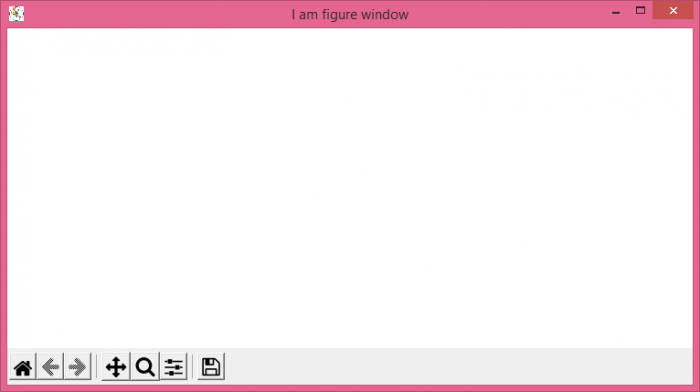# What's the difference between Matplotlib.pyplot and Matplotlib.figure?

## matplotlib.pyplot

The matplotlib.pyplot is a collection of functions that make matplotlib work like MATLAB. Each pyplot function makes some change to a figure: e.g., creates a figure, creates a plotting area in a figure, plots some lines in a plotting area, decorates the plot with labels, etc.

In matplotlib.pyplot, various states are preserved across function calls, so that it keeps track of things like the current figure and plotting area, and the plotting functions are directed to the current axes

## matplotlib.figure

The figure keeps track of all the child Axes, a smattering of 'special' artists (titles, figure legends,etc), and the canvas. A figure can contain any number of Axes, but will typically have at least one.

## Example

from matplotlib import pyplot as plt

plt.rcParams["figure.figsize"] = [7.50, 3.50]
plt.rcParams["figure.autolayout"] = True

fig = plt.figure("I am figure window")

plt.show()

## Output